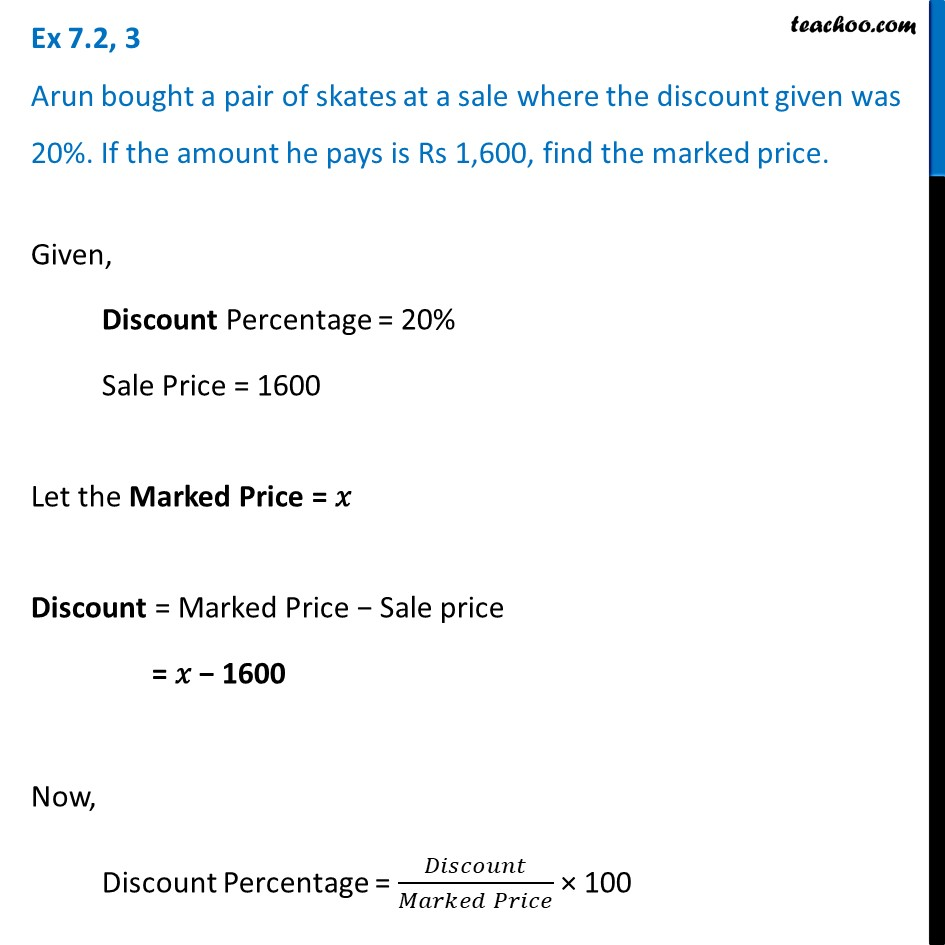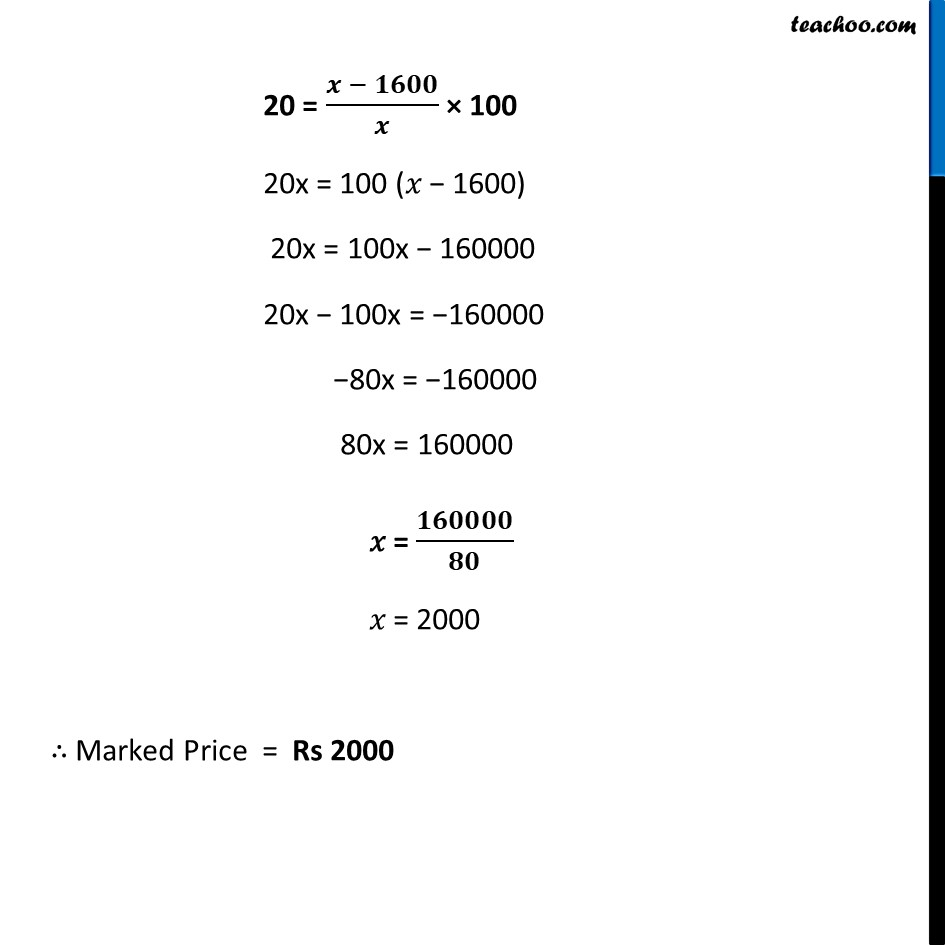Ex 7.2

Chapter 7 Class 8 Comparing Quantities
Serial order wiseLearn in your speed, with individual attention - Teachoo Maths 1-on-1 Class

### Transcript

Ex 7.2, 3 Arun bought a pair of skates at a sale where the discount given was 20%. If the amount he pays is Rs 1,600, find the marked price.Given, Discount Percentage = 20% Sale Price = 1600 Let the Marked Price = 𝑥 Discount = Marked Price − Sale price = 𝑥 − 1600 Now, Discount Percentage = 𝐷𝑖𝑠𝑐𝑜𝑢𝑛𝑡/(𝑀𝑎𝑟𝑘𝑒𝑑 𝑃𝑟𝑖𝑐𝑒) × 100 20 = (𝒙 − 𝟏𝟔𝟎𝟎)/𝒙 × 100 20x = 100 (𝑥 − 1600) 20x = 100x − 160000 20x − 100x = −160000 −80x = −160000 80x = 160000 𝑥 = 𝟏𝟔𝟎𝟎𝟎𝟎/𝟖𝟎 𝑥 = 2000 ∴ Marked Price = Rs 2000